Скачать презентацию CPSC 689 Discrete Algorithms for Mobile and Wireless

• Количество слайдов: 34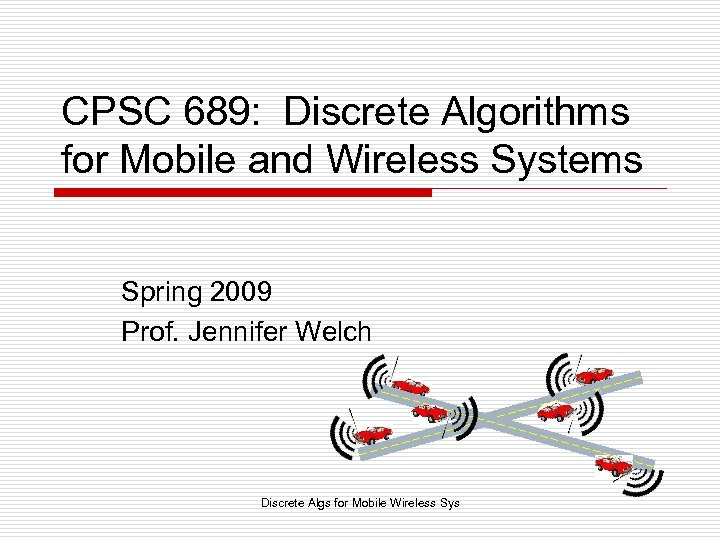CPSC 689: Discrete Algorithms for Mobile and Wireless Systems Spring 2009 Prof. Jennifer Welch Discrete Algs for Mobile Wireless Sys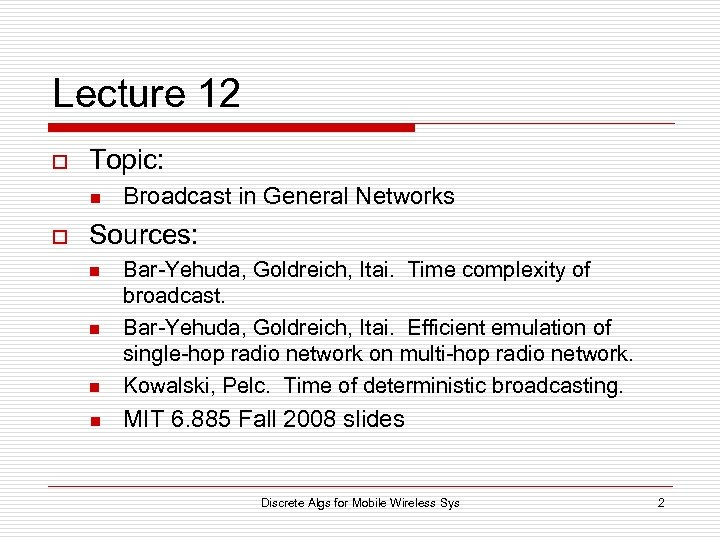Lecture 12 o Topic: n o Broadcast in General Networks Sources: n Bar-Yehuda, Goldreich, Itai. Time complexity of broadcast. Bar-Yehuda, Goldreich, Itai. Efficient emulation of single-hop radio network on multi-hop radio network. Kowalski, Pelc. Time of deterministic broadcasting. n MIT 6. 885 Fall 2008 slides n n Discrete Algs for Mobile Wireless Sys 2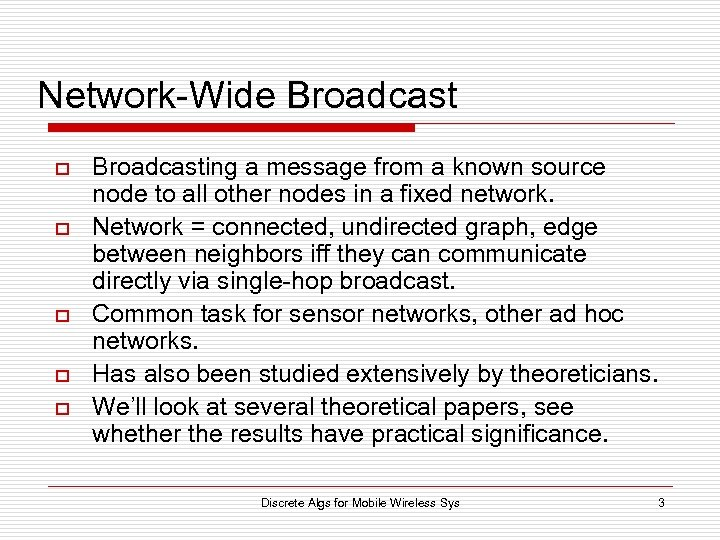Network-Wide Broadcast o o o Broadcasting a message from a known source node to all other nodes in a fixed network. Network = connected, undirected graph, edge between neighbors iff they can communicate directly via single-hop broadcast. Common task for sensor networks, other ad hoc networks. Has also been studied extensively by theoreticians. We’ll look at several theoretical papers, see whether the results have practical significance. Discrete Algs for Mobile Wireless Sys 3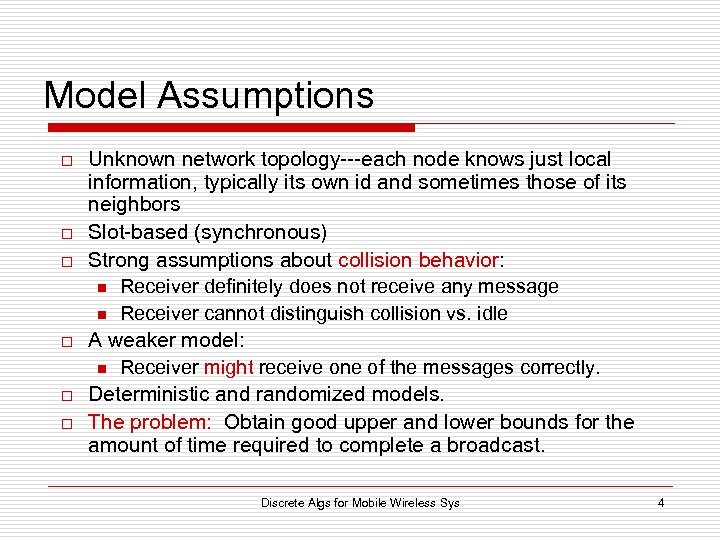Model Assumptions o o o Unknown network topology---each node knows just local information, typically its own id and sometimes those of its neighbors Slot-based (synchronous) Strong assumptions about collision behavior: n Receiver definitely does not receive any message n Receiver cannot distinguish collision vs. idle A weaker model: n Receiver might receive one of the messages correctly. Deterministic and randomized models. The problem: Obtain good upper and lower bounds for the amount of time required to complete a broadcast. Discrete Algs for Mobile Wireless Sys 4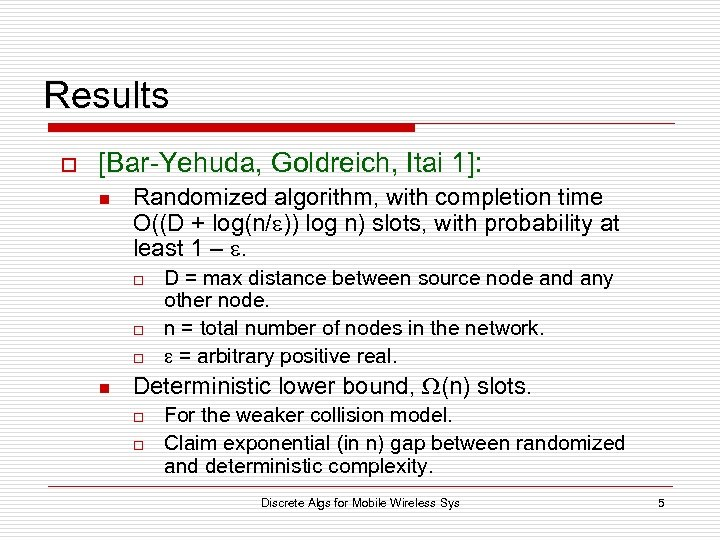Results o [Bar-Yehuda, Goldreich, Itai 1]: n Randomized algorithm, with completion time O((D + log(n/ )) log n) slots, with probability at least 1 – . o o o n D = max distance between source node and any other node. n = total number of nodes in the network. = arbitrary positive real. Deterministic lower bound, (n) slots. o o For the weaker collision model. Claim exponential (in n) gap between randomized and deterministic complexity. Discrete Algs for Mobile Wireless Sys 5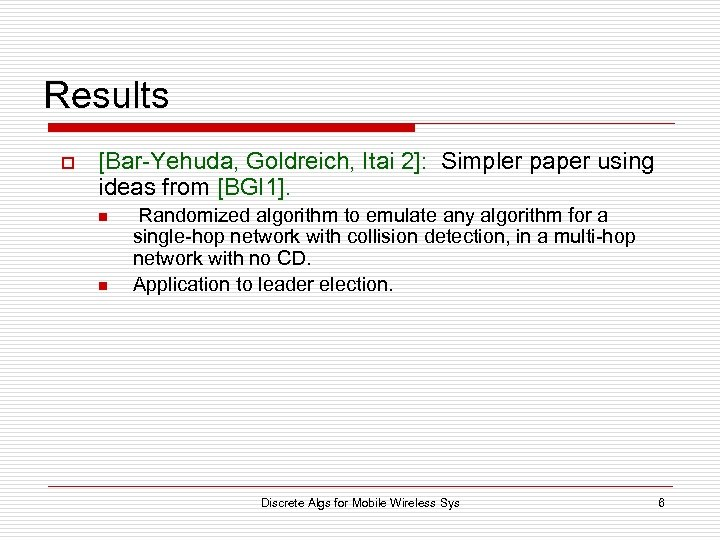Results o [Bar-Yehuda, Goldreich, Itai 2]: Simpler paper using ideas from [BGI 1]. n n Randomized algorithm to emulate any algorithm for a single-hop network with collision detection, in a multi-hop network with no CD. Application to leader election. Discrete Algs for Mobile Wireless Sys 6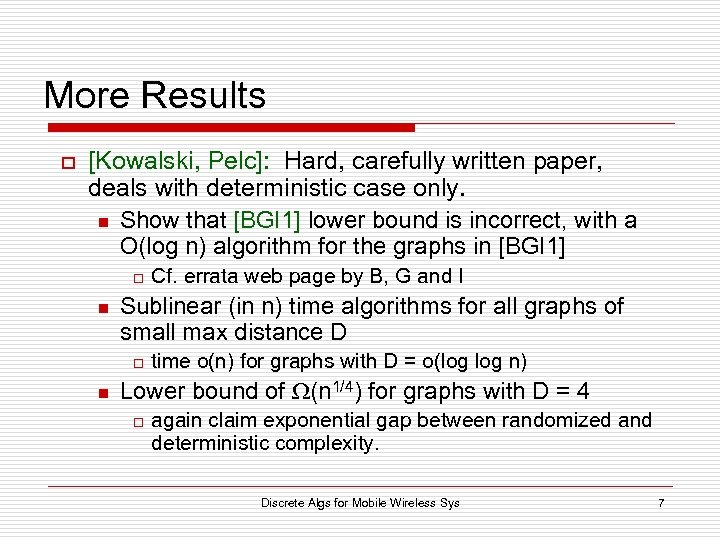More Results o [Kowalski, Pelc]: Hard, carefully written paper, deals with deterministic case only. n Show that [BGI 1] lower bound is incorrect, with a O(log n) algorithm for the graphs in [BGI 1] o n Sublinear (in n) time algorithms for all graphs of small max distance D o n Cf. errata web page by B, G and I time o(n) for graphs with D = o(log n) Lower bound of (n 1/4) for graphs with D = 4 o again claim exponential gap between randomized and deterministic complexity. Discrete Algs for Mobile Wireless Sys 7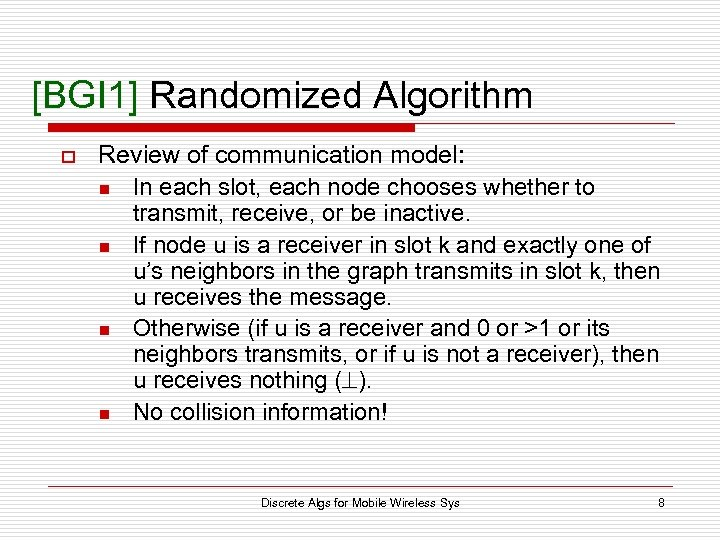[BGI 1] Randomized Algorithm o Review of communication model: n In each slot, each node chooses whether to transmit, receive, or be inactive. n If node u is a receiver in slot k and exactly one of u’s neighbors in the graph transmits in slot k, then u receives the message. n Otherwise (if u is a receiver and 0 or >1 or its neighbors transmits, or if u is not a receiver), then u receives nothing ( ). n No collision information! Discrete Algs for Mobile Wireless Sys 8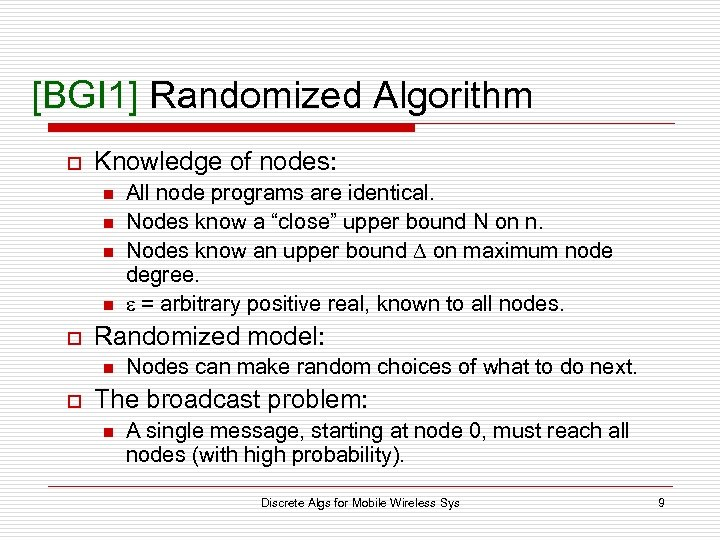[BGI 1] Randomized Algorithm o Knowledge of nodes: n n o Randomized model: n o All node programs are identical. Nodes know a “close” upper bound N on n. Nodes know an upper bound on maximum node degree. = arbitrary positive real, known to all nodes. Nodes can make random choices of what to do next. The broadcast problem: n A single message, starting at node 0, must reach all nodes (with high probability). Discrete Algs for Mobile Wireless Sys 9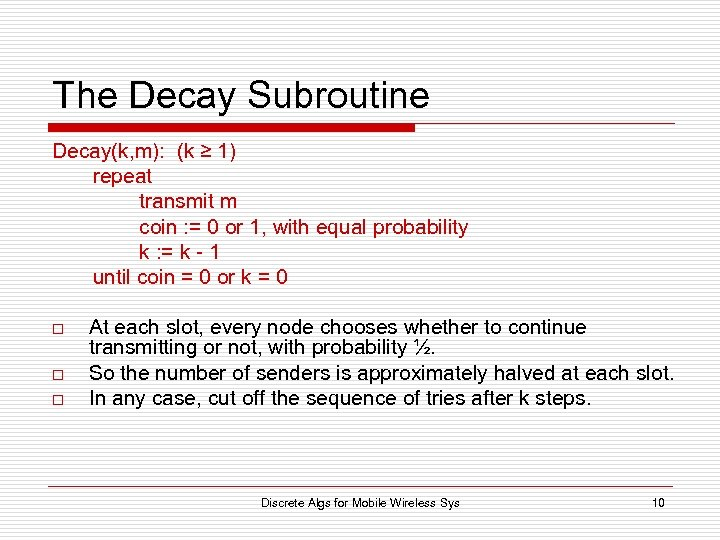The Decay Subroutine Decay(k, m): (k ≥ 1) repeat transmit m coin : = 0 or 1, with equal probability k : = k - 1 until coin = 0 or k = 0 o o o At each slot, every node chooses whether to continue transmitting or not, with probability ½. So the number of senders is approximately halved at each slot. In any case, cut off the sequence of tries after k steps. Discrete Algs for Mobile Wireless Sys 10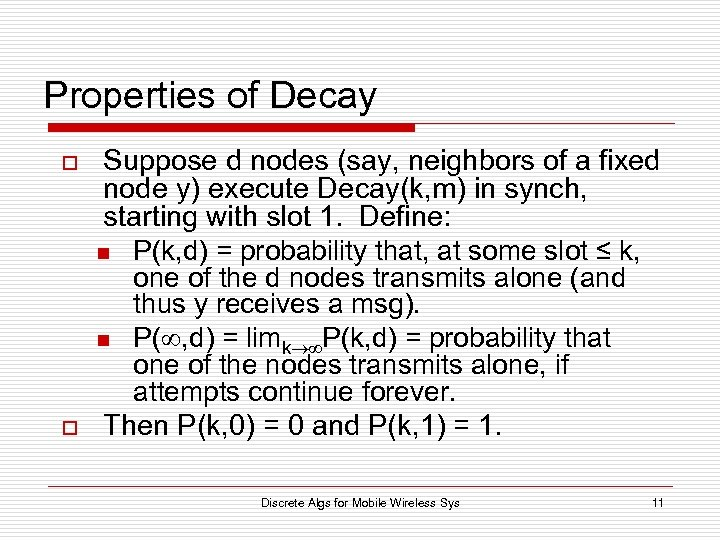Properties of Decay o o Suppose d nodes (say, neighbors of a fixed node y) execute Decay(k, m) in synch, starting with slot 1. Define: n P(k, d) = probability that, at some slot ≤ k, one of the d nodes transmits alone (and thus y receives a msg). n P( , d) = limk P(k, d) = probability that one of the nodes transmits alone, if attempts continue forever. Then P(k, 0) = 0 and P(k, 1) = 1. Discrete Algs for Mobile Wireless Sys 11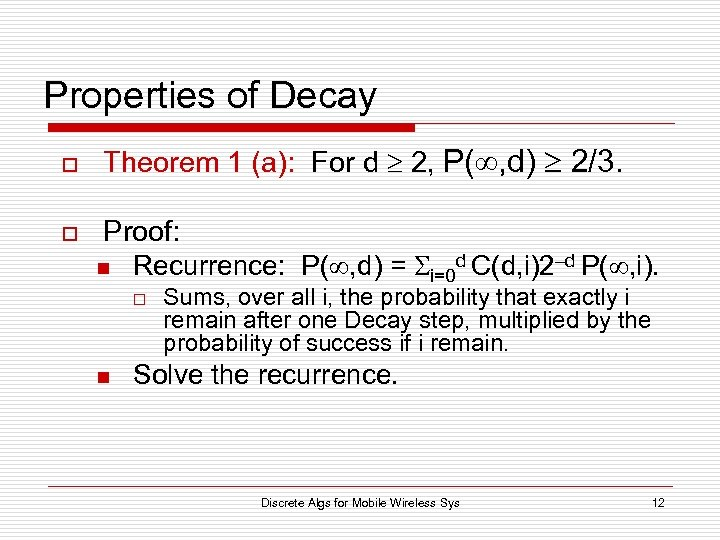Properties of Decay o o Theorem 1 (a): For d 2, P( , d) 2/3. Proof: n Recurrence: P( , d) = i=0 d C(d, i)2–d P( , i). o n Sums, over all i, the probability that exactly i remain after one Decay step, multiplied by the probability of success if i remain. Solve the recurrence. Discrete Algs for Mobile Wireless Sys 12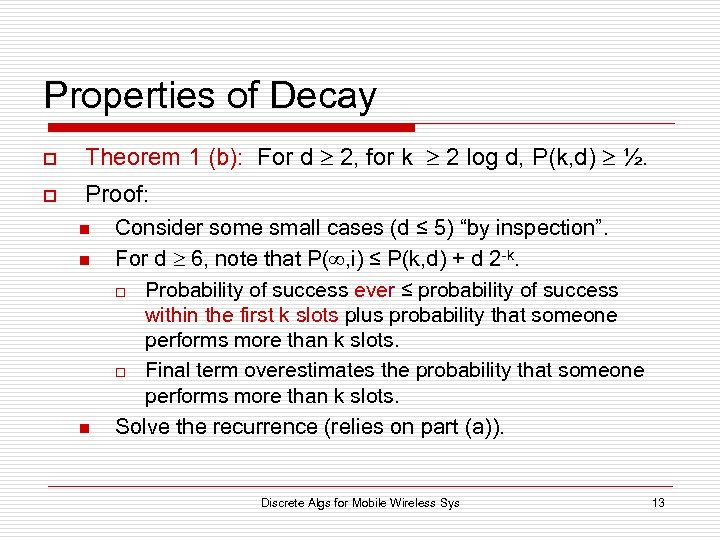Properties of Decay o Theorem 1 (b): For d 2, for k 2 log d, P(k, d) ½. o Proof: n n n Consider some small cases (d ≤ 5) “by inspection”. For d 6, note that P( , i) ≤ P(k, d) + d 2 -k. o Probability of success ever ≤ probability of success within the first k slots plus probability that someone performs more than k slots. o Final term overestimates the probability that someone performs more than k slots. Solve the recurrence (relies on part (a)). Discrete Algs for Mobile Wireless Sys 13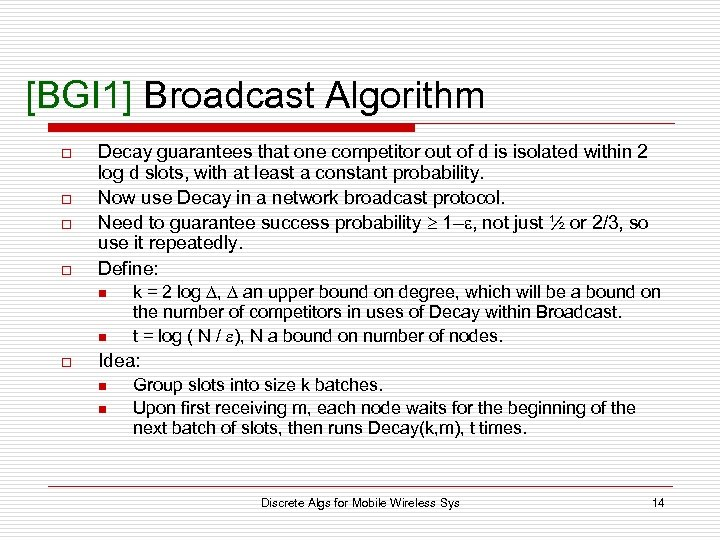[BGI 1] Broadcast Algorithm o o Decay guarantees that one competitor out of d is isolated within 2 log d slots, with at least a constant probability. Now use Decay in a network broadcast protocol. Need to guarantee success probability 1– , not just ½ or 2/3, so use it repeatedly. Define: n n o k = 2 log , an upper bound on degree, which will be a bound on the number of competitors in uses of Decay within Broadcast. t = log ( N / ), N a bound on number of nodes. Idea: n n Group slots into size k batches. Upon first receiving m, each node waits for the beginning of the next batch of slots, then runs Decay(k, m), t times. Discrete Algs for Mobile Wireless Sys 14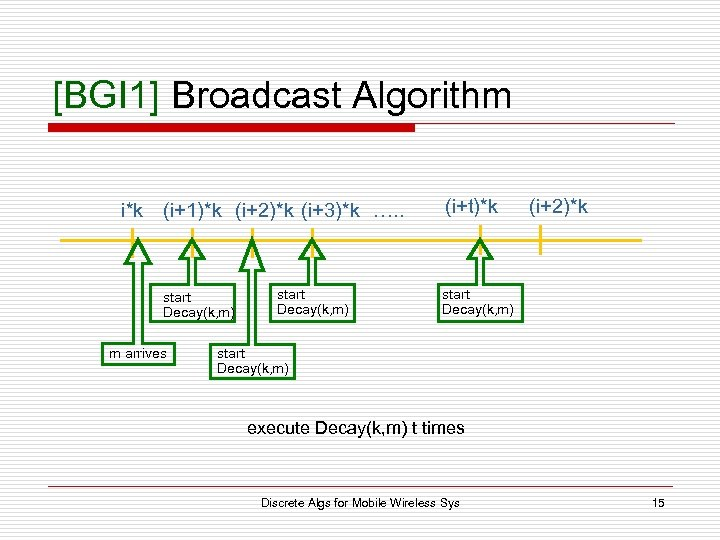[BGI 1] Broadcast Algorithm i*k (i+1)*k (i+2)*k (i+3)*k …. . start Decay(k, m) m arrives start Decay(k, m) (i+t)*k (i+2)*k start Decay(k, m) execute Decay(k, m) t times Discrete Algs for Mobile Wireless Sys 15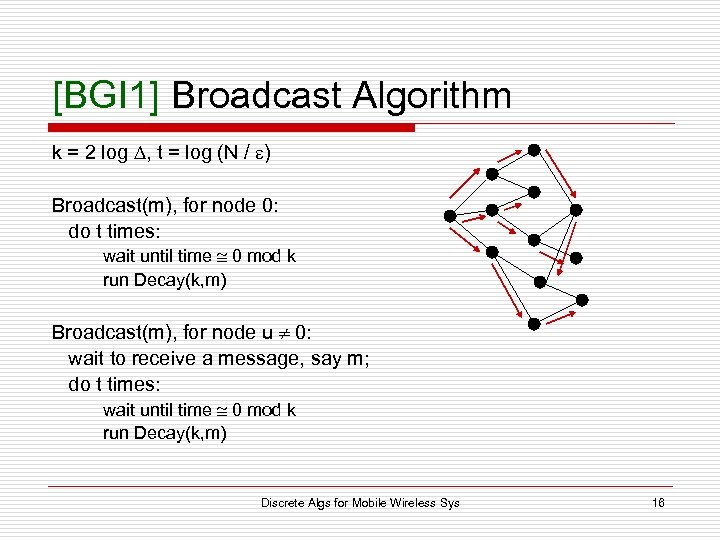[BGI 1] Broadcast Algorithm k = 2 log , t = log (N / ) Broadcast(m), for node 0: do t times: wait until time 0 mod k run Decay(k, m) Broadcast(m), for node u 0: wait to receive a message, say m; do t times: wait until time 0 mod k run Decay(k, m) Discrete Algs for Mobile Wireless Sys 16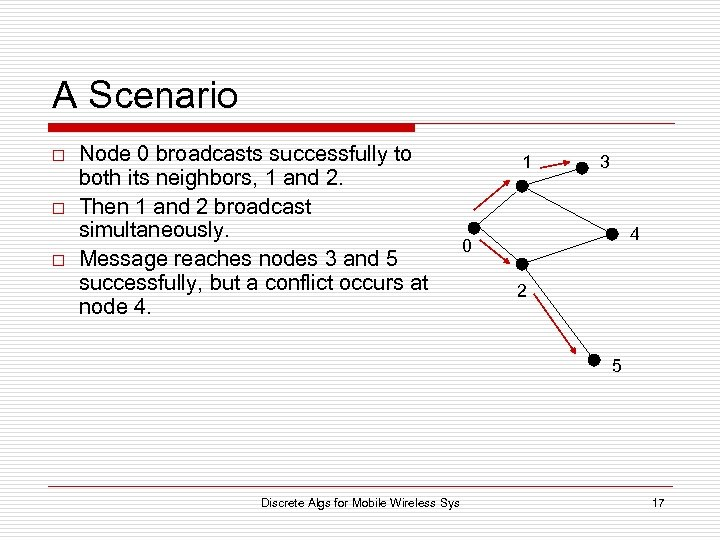A Scenario o Node 0 broadcasts successfully to both its neighbors, 1 and 2. Then 1 and 2 broadcast simultaneously. Message reaches nodes 3 and 5 successfully, but a conflict occurs at node 4. 1 3 4 0 2 5 Discrete Algs for Mobile Wireless Sys 17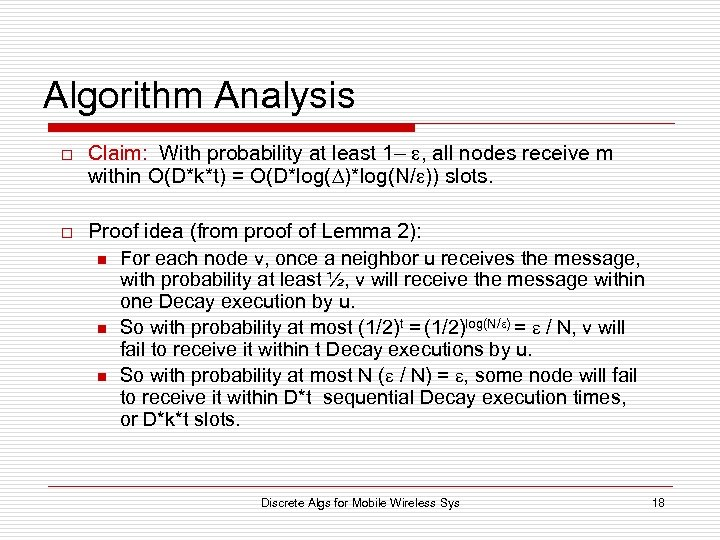Algorithm Analysis o o Claim: With probability at least 1– , all nodes receive m within O(D*k*t) = O(D*log( )*log(N/ )) slots. Proof idea (from proof of Lemma 2): n For each node v, once a neighbor u receives the message, with probability at least ½, v will receive the message within one Decay execution by u. n So with probability at most (1/2)t = (1/2)log(N/ ) = / N, v will fail to receive it within t Decay executions by u. n So with probability at most N ( / N) = , some node will fail to receive it within D*t sequential Decay execution times, or D*k*t slots. Discrete Algs for Mobile Wireless Sys 18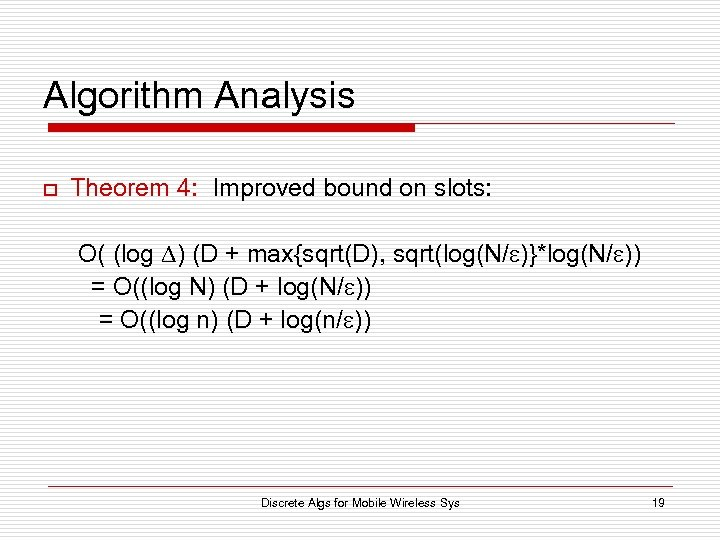Algorithm Analysis o Theorem 4: Improved bound on slots: O( (log ) (D + max{sqrt(D), sqrt(log(N/ )}*log(N/ )) = O((log N) (D + log(N/ )) = O((log n) (D + log(n/ )) Discrete Algs for Mobile Wireless Sys 19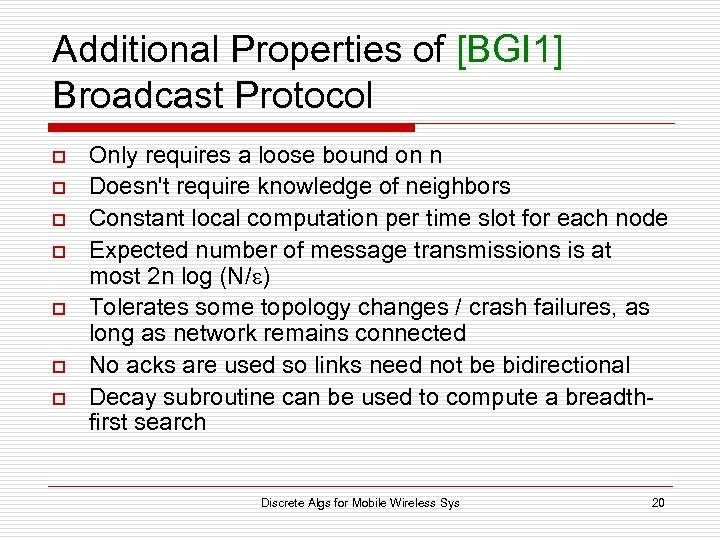Additional Properties of [BGI 1] Broadcast Protocol o o o o Only requires a loose bound on n Doesn't require knowledge of neighbors Constant local computation per time slot for each node Expected number of message transmissions is at most 2 n log (N/ ) Tolerates some topology changes / crash failures, as long as network remains connected No acks are used so links need not be bidirectional Decay subroutine can be used to compute a breadthfirst search Discrete Algs for Mobile Wireless Sys 20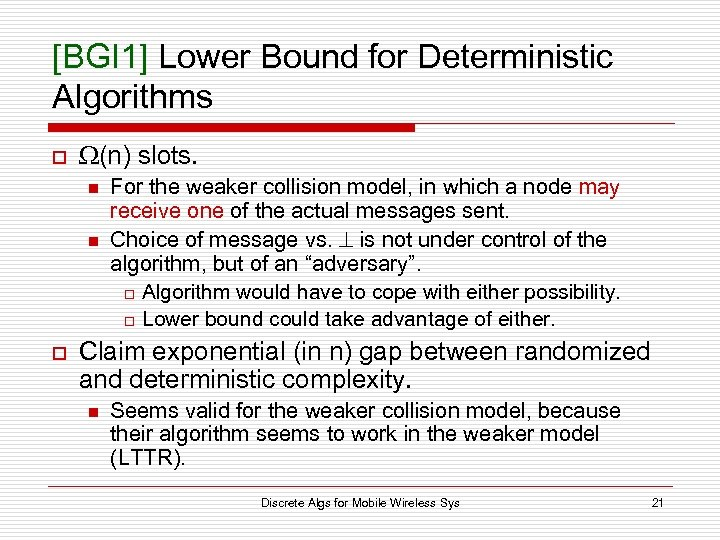[BGI 1] Lower Bound for Deterministic Algorithms o (n) slots. n n o For the weaker collision model, in which a node may receive one of the actual messages sent. Choice of message vs. is not under control of the algorithm, but of an “adversary”. o Algorithm would have to cope with either possibility. o Lower bound could take advantage of either. Claim exponential (in n) gap between randomized and deterministic complexity. n Seems valid for the weaker collision model, because their algorithm seems to work in the weaker model (LTTR). Discrete Algs for Mobile Wireless Sys 21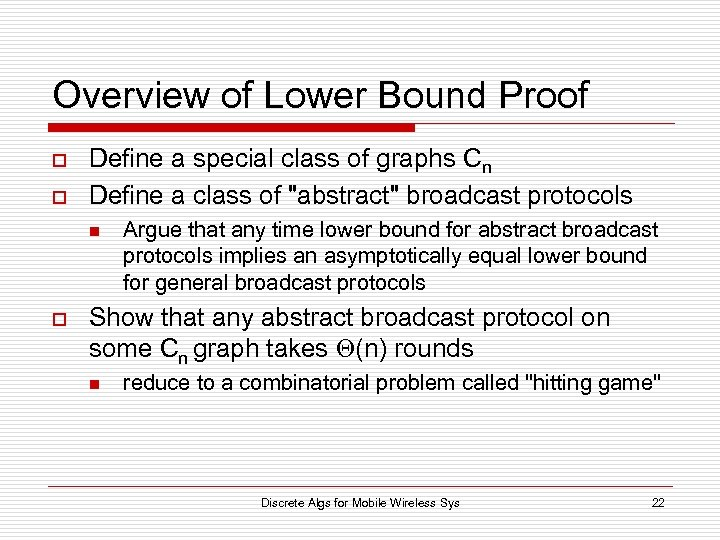Overview of Lower Bound Proof o o Define a special class of graphs Cn Define a class of "abstract" broadcast protocols n o Argue that any time lower bound for abstract broadcast protocols implies an asymptotically equal lower bound for general broadcast protocols Show that any abstract broadcast protocol on some Cn graph takes (n) rounds n reduce to a combinatorial problem called "hitting game" Discrete Algs for Mobile Wireless Sys 22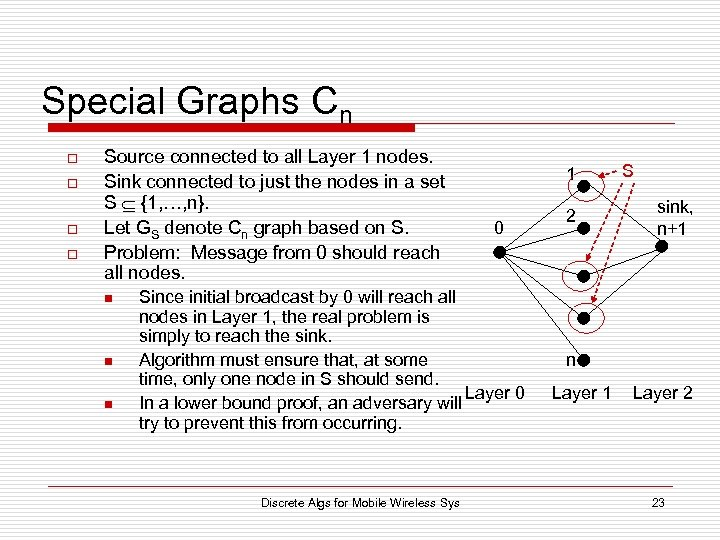Special Graphs Cn o o Source connected to all Layer 1 nodes. Sink connected to just the nodes in a set S {1, …, n}. Let GS denote Cn graph based on S. Problem: Message from 0 should reach all nodes. n n n 1 0 Since initial broadcast by 0 will reach all nodes in Layer 1, the real problem is simply to reach the sink. Algorithm must ensure that, at some time, only one node in S should send. Layer 0 In a lower bound proof, an adversary will try to prevent this from occurring. Discrete Algs for Mobile Wireless Sys 2 S sink, n+1 n Layer 1 Layer 2 23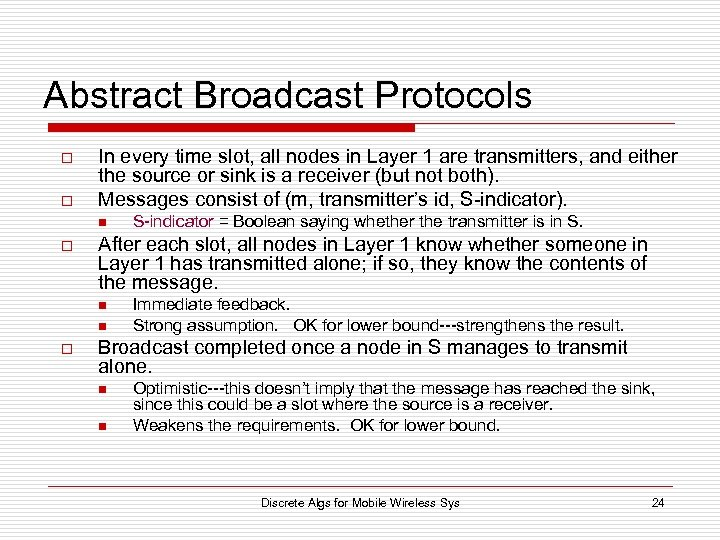Abstract Broadcast Protocols o o In every time slot, all nodes in Layer 1 are transmitters, and either the source or sink is a receiver (but not both). Messages consist of (m, transmitter’s id, S-indicator). n o After each slot, all nodes in Layer 1 know whether someone in Layer 1 has transmitted alone; if so, they know the contents of the message. n n o S-indicator = Boolean saying whether the transmitter is in S. Immediate feedback. Strong assumption. OK for lower bound---strengthens the result. Broadcast completed once a node in S manages to transmit alone. n n Optimistic---this doesn’t imply that the message has reached the sink, since this could be a slot where the source is a receiver. Weakens the requirements. OK for lower bound. Discrete Algs for Mobile Wireless Sys 24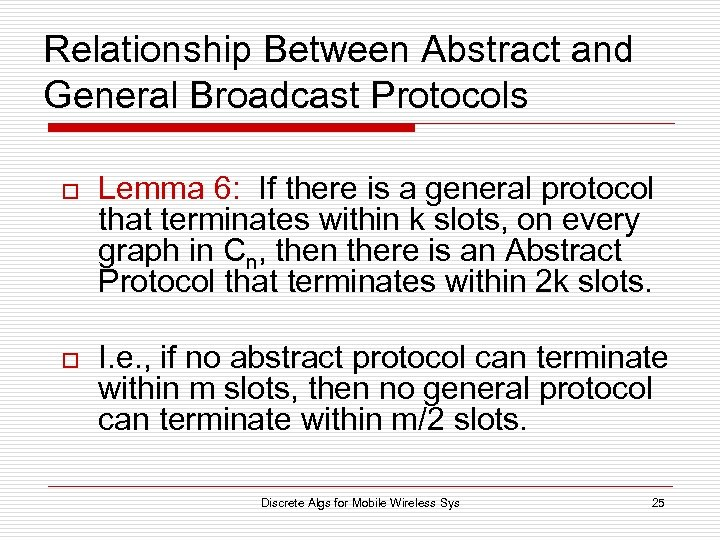Relationship Between Abstract and General Broadcast Protocols o Lemma 6: If there is a general protocol that terminates within k slots, on every graph in Cn, then there is an Abstract Protocol that terminates within 2 k slots. o I. e. , if no abstract protocol can terminate within m slots, then no general protocol can terminate within m/2 slots. Discrete Algs for Mobile Wireless Sys 25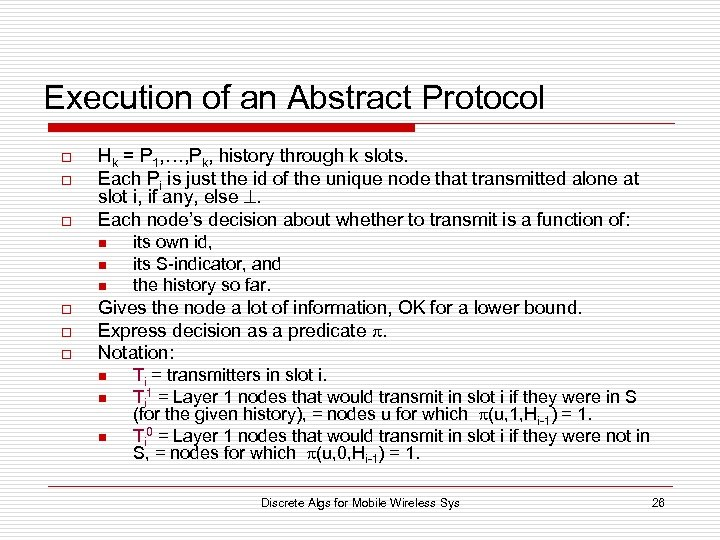Execution of an Abstract Protocol o o o Hk = P 1, …, Pk, history through k slots. Each Pi is just the id of the unique node that transmitted alone at slot i, if any, else . Each node’s decision about whether to transmit is a function of: n n n o o o its own id, its S-indicator, and the history so far. Gives the node a lot of information, OK for a lower bound. Express decision as a predicate . Notation: n n n Ti = transmitters in slot i. Ti 1 = Layer 1 nodes that would transmit in slot i if they were in S (for the given history), = nodes u for which (u, 1, Hi-1) = 1. Ti 0 = Layer 1 nodes that would transmit in slot i if they were not in S, = nodes for which (u, 0, Hi-1) = 1. Discrete Algs for Mobile Wireless Sys 26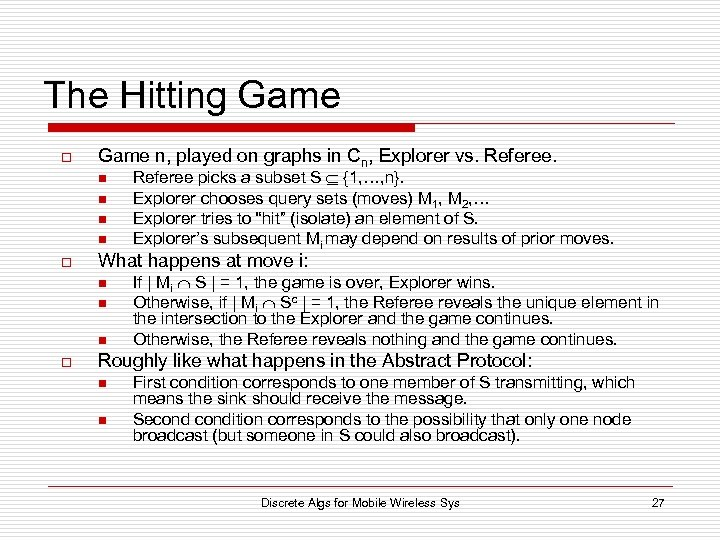The Hitting Game o Game n, played on graphs in Cn, Explorer vs. Referee. n n o What happens at move i: n n n o Referee picks a subset S {1, …, n}. Explorer chooses query sets (moves) M 1, M 2, … Explorer tries to “hit” (isolate) an element of S. Explorer’s subsequent Mi may depend on results of prior moves. If | Mi S | = 1, the game is over, Explorer wins. Otherwise, if | Mi Sc | = 1, the Referee reveals the unique element in the intersection to the Explorer and the game continues. Otherwise, the Referee reveals nothing and the game continues. Roughly like what happens in the Abstract Protocol: n n First condition corresponds to one member of S transmitting, which means the sink should receive the message. Secondition corresponds to the possibility that only one node broadcast (but someone in S could also broadcast). Discrete Algs for Mobile Wireless Sys 27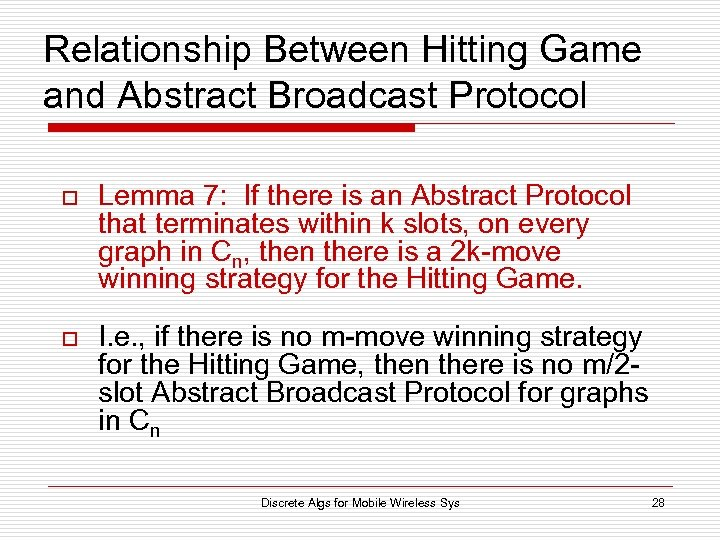Relationship Between Hitting Game and Abstract Broadcast Protocol o Lemma 7: If there is an Abstract Protocol that terminates within k slots, on every graph in Cn, then there is a 2 k-move winning strategy for the Hitting Game. o I. e. , if there is no m-move winning strategy for the Hitting Game, then there is no m/2 slot Abstract Broadcast Protocol for graphs in Cn Discrete Algs for Mobile Wireless Sys 28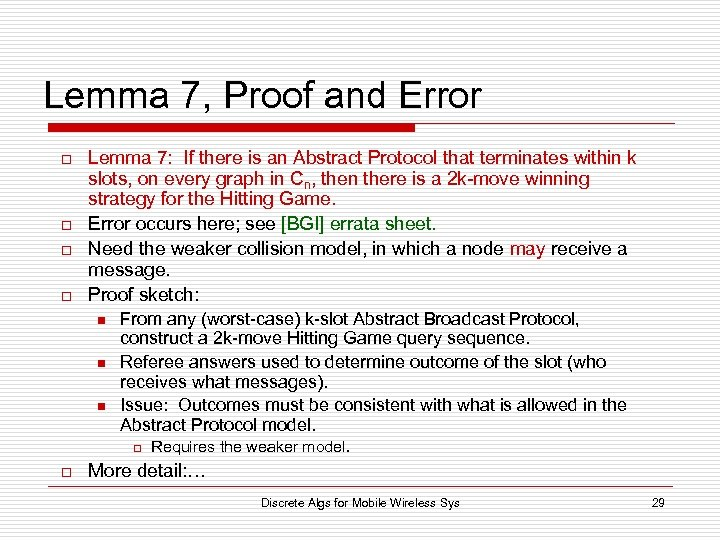Lemma 7, Proof and Error o o Lemma 7: If there is an Abstract Protocol that terminates within k slots, on every graph in Cn, then there is a 2 k-move winning strategy for the Hitting Game. Error occurs here; see [BGI] errata sheet. Need the weaker collision model, in which a node may receive a message. Proof sketch: n n n From any (worst-case) k-slot Abstract Broadcast Protocol, construct a 2 k-move Hitting Game query sequence. Referee answers used to determine outcome of the slot (who receives what messages). Issue: Outcomes must be consistent with what is allowed in the Abstract Protocol model. o o Requires the weaker model. More detail: … Discrete Algs for Mobile Wireless Sys 29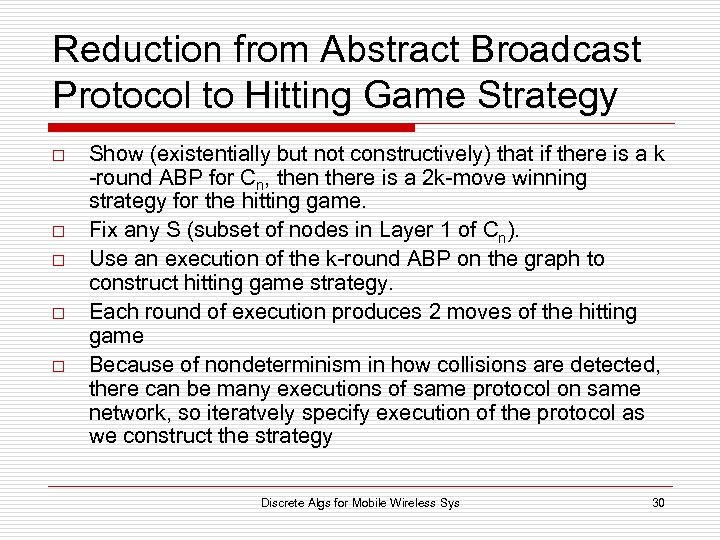Reduction from Abstract Broadcast Protocol to Hitting Game Strategy o o o Show (existentially but not constructively) that if there is a k -round ABP for Cn, then there is a 2 k-move winning strategy for the hitting game. Fix any S (subset of nodes in Layer 1 of Cn). Use an execution of the k-round ABP on the graph to construct hitting game strategy. Each round of execution produces 2 moves of the hitting game Because of nondeterminism in how collisions are detected, there can be many executions of same protocol on same network, so iteratvely specify execution of the protocol as we construct the strategy Discrete Algs for Mobile Wireless Sys 30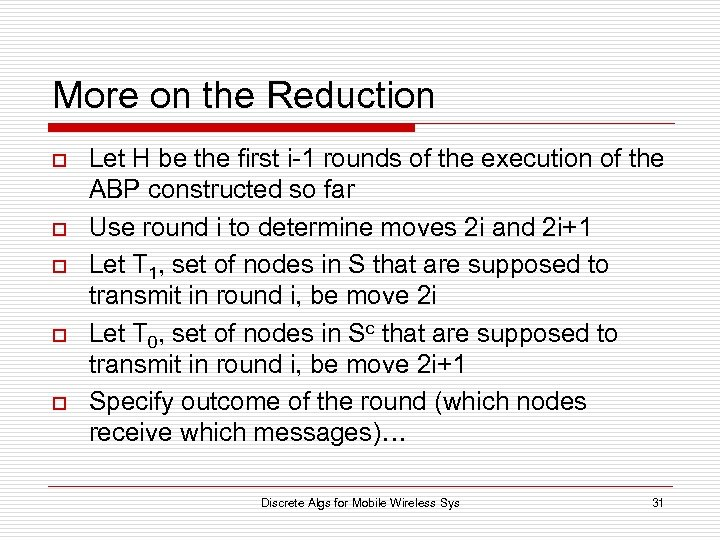More on the Reduction o o o Let H be the first i-1 rounds of the execution of the ABP constructed so far Use round i to determine moves 2 i and 2 i+1 Let T 1, set of nodes in S that are supposed to transmit in round i, be move 2 i Let T 0, set of nodes in Sc that are supposed to transmit in round i, be move 2 i+1 Specify outcome of the round (which nodes receive which messages)… Discrete Algs for Mobile Wireless Sys 31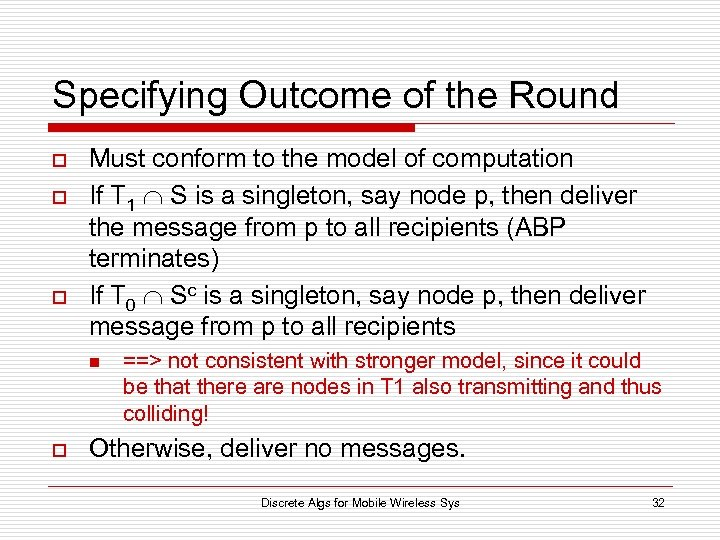Specifying Outcome of the Round o o o Must conform to the model of computation If T 1 S is a singleton, say node p, then deliver the message from p to all recipients (ABP terminates) If T 0 Sc is a singleton, say node p, then deliver message from p to all recipients n o ==> not consistent with stronger model, since it could be that there are nodes in T 1 also transmitting and thus colliding! Otherwise, deliver no messages. Discrete Algs for Mobile Wireless Sys 32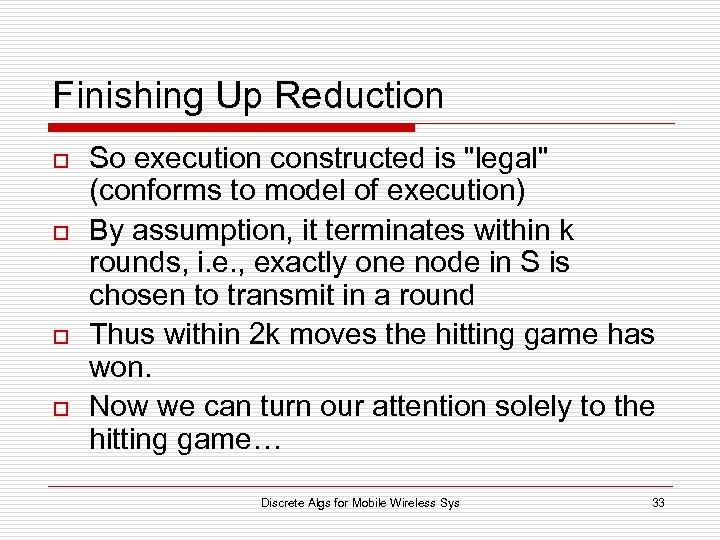Finishing Up Reduction o o So execution constructed is "legal" (conforms to model of execution) By assumption, it terminates within k rounds, i. e. , exactly one node in S is chosen to transmit in a round Thus within 2 k moves the hitting game has won. Now we can turn our attention solely to the hitting game… Discrete Algs for Mobile Wireless Sys 33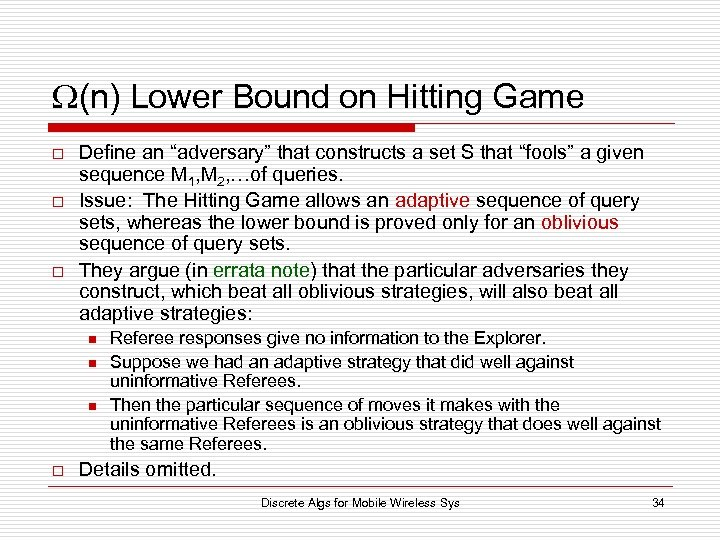(n) Lower Bound on Hitting Game o o o Define an “adversary” that constructs a set S that “fools” a given sequence M 1, M 2, …of queries. Issue: The Hitting Game allows an adaptive sequence of query sets, whereas the lower bound is proved only for an oblivious sequence of query sets. They argue (in errata note) that the particular adversaries they construct, which beat all oblivious strategies, will also beat all adaptive strategies: n n n o Referee responses give no information to the Explorer. Suppose we had an adaptive strategy that did well against uninformative Referees. Then the particular sequence of moves it makes with the uninformative Referees is an oblivious strategy that does well against the same Referees. Details omitted. Discrete Algs for Mobile Wireless Sys 34